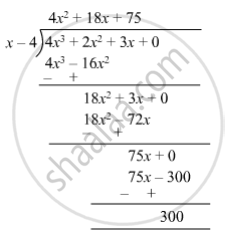SSC (English Medium) Class 8Maharashtra State Board
Share

# Divide and Write the Quotient and the Remainder. (3x + 2x2 + 4x3) ÷ (X − 4) - SSC (English Medium) Class 8 - Mathematics

ConceptTo Divide a Polynomial by a Binomial

#### Question

Divide and write the quotient and the remainder.

(3x + 2x2 + 4x3) ÷ (x − 4)

#### Solution

(3x + 2x2 + 4x3) ÷ (x − 4)So, quotient = $4 x^2 + 18x + 75$  and remainder = 300.

Is there an error in this question or solution?

#### APPEARS IN

Balbharati Solution for Balbharati Class 8 Mathematics (2019 to Current)
Chapter 10: Division of Polynomials
Practice Set 10.2 | Q: 3 | Page no. 66
Solution Divide and Write the Quotient and the Remainder. (3x + 2x2 + 4x3) ÷ (X − 4) Concept: To Divide a Polynomial by a Binomial.
S